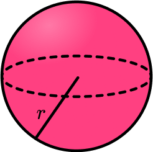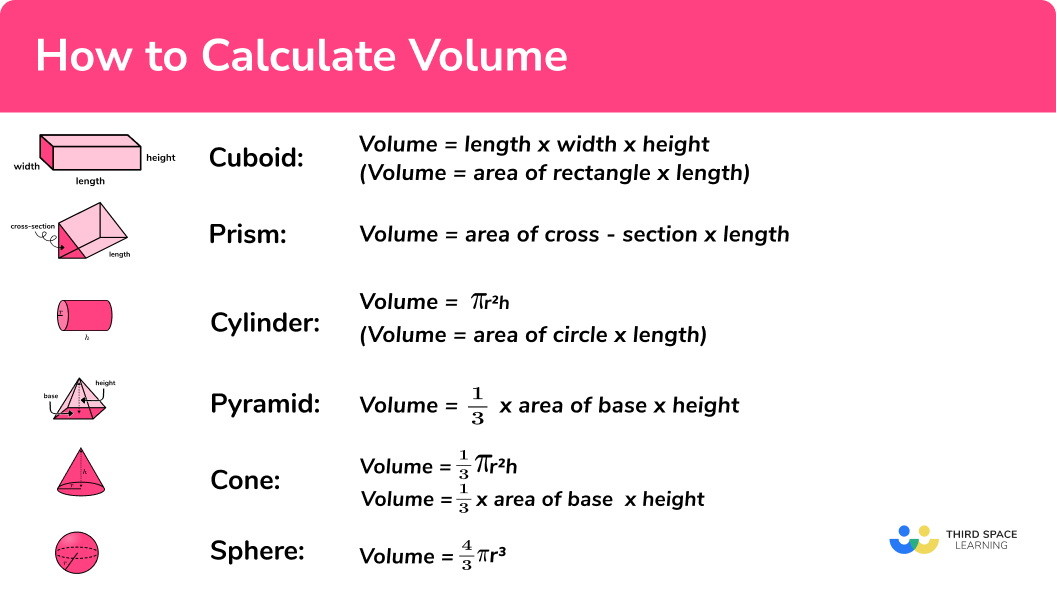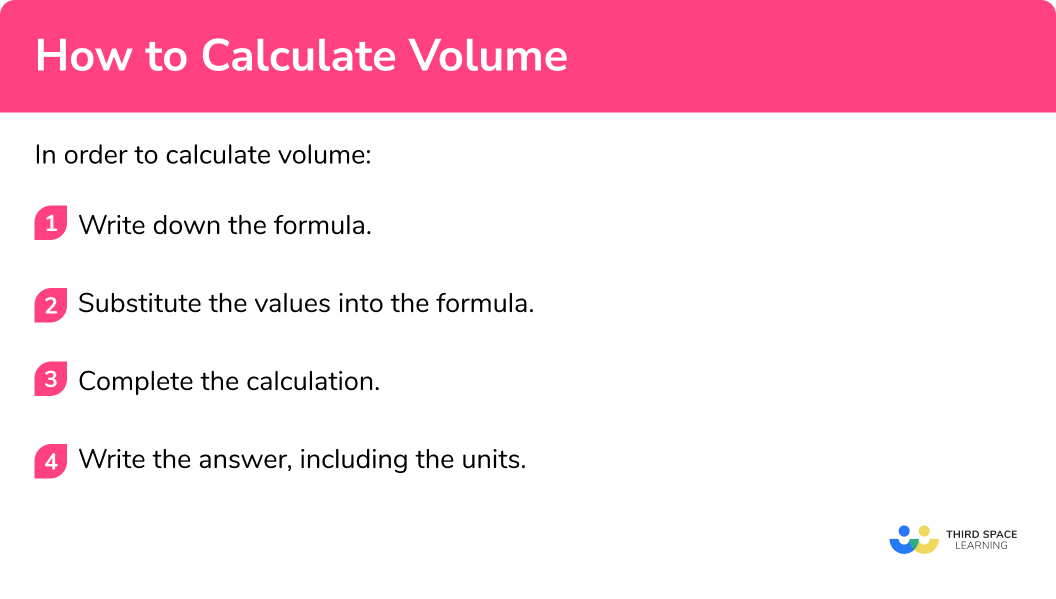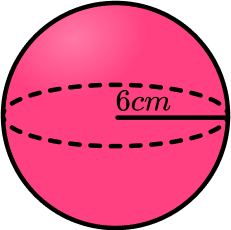GCSE Maths Geometry and Measure

Volume

How to Calculate Volume

# How to Calculate Volume

Here we will learn how to calculate the volume of three-dimensional shapes, including cubes/cuboids, prisms, cylinders, pyramids, cones and spheres.

There are also volume worksheets based on Edexcel, AQA and OCR exam questions, along with further guidance on where to go next if you’re still stuck.

## What is volume?

The volume of a 3 D shape is the amount of space there is inside of the shape.

To calculate the volume of a shape in three dimensions, we can use the relevant volume formula.

The table below shows the formulae that we can use for some of the most common 3D shapes:

CuboidPrismCylinderPyramidConeSphere\text{Volume }={h}\times{w}\times{d}

(\text{Volume }=
\text{Area of rectangle } \times \text{ Depth})

\text{Volume }=
\text{Area of cross section} \times \text{ Depth}

\text{Volume }=\pi{r}^2{h}

(\text{Volume }=
\text{Area of circle }\times\text{ Height})

\text{Volume }=
\frac{1}{3}\times\text{Area of base }\times\text{Height}

\text{Volume }=\frac{1}{3}\pi{r}^2{h}

\text{Volume }=
\frac{1}{3}\times\text{Area of base }\times\text{Height}

\text{Volume }=\frac{4}{3}\pi{r}^3

The formulae for the volume of a cone and the volume of a sphere are given to you for GCSE maths. You need to learn the other formulae.

Volume is measured in metric units, including cubic units such as cm^3 (cubic centimetres), m^3 (cubic metres) or mm^3 (cubic millimetres). Volume could also be measured in imperial units, including cubic feet or cubic inches.

### What is volume?## Volume of composite solid

You may be asked to calculate the volume of a 3 dimensional shape which is composed of 2 or more separate shapes. This type of shape is known as a composite shape. The volume of each of the shapes can be found and then added together.

For example,

Here is a composite shape. It is made of a cuboid and a pyramid.

The volume of the cuboid is

8\times 8\times 10=640

The volume of the pyramid is

\frac{1}{3} \times 8^2 \times 6=128

The total volume can be found by adding the volume of the cuboid and the volume of the pyramid.

640+128=768 \ cm^3

## How to calculate volume

In order to calculate volume:

1. Write down the formula.
2. Substitute the values into the formula.
3. Complete the calculation.
4. Write the answer, including the units.

### Explain how to calculate volume## Volume examples

### Example 1: volume of a cuboid

Calculate the volume of the cuboid below:

1. Write down the formula.

The volume (V) of a cuboid is the same as the volume of a rectangular prism or the volume of a box.

\text{Volume } = {h}\times{w}\times{d}

2Substitute the values into the formula.

Given that h=3cm, \; w= 9cm, and d= 5cm, we have:

V = 3 \times 9 \times 5

3Complete the calculation.

V = 135

4Write the answer, including the units.

The dimensions of the cuboid are given in centimetres, so the volume will be in cubic centimetres (cm^3) .

V = 135cm^3

### Example 2: volume of a prism

Calculate the volume of this triangular prism:

\text{Volume of prism } = \text{Area of cross section} \times \text{Depth}

First, we need to find the area of the cross section, which is a triangle:

\begin{aligned} \text{Area of a triangle}&=\frac{1}{2}\times b \times h\\\\ &=\frac{1}{2}\times 2 \times 7\\\\ &=7\text{ cm}^2 \end{aligned}

The depth of the prism is 6m, therefore:

\begin{aligned} \text{Volume of prism }&= \text{Area of cross section} \times \text{ Depth}\\\\ &=7\times 6 \end{aligned}

V = 42

The dimensions are given in metres, so the volume is given in cubic metres (m3):

V=42m^3

### Example 3: volume of a cylinder

Find the volume of the cylinder. Give your answer to 2 decimal places.

\text{Volume of a cylinder} = \pi r^{2}h

The height of the cylinder is 7cm and the radius is 5cm.

V=\pi \times 5^{2} \times 7

V=\pi \times 5^{2} \times 7 = 549.7787144...

V = 549.78cm^3

### Example 4: volume of a pyramid

Work out the volume of the pyramid:

\text{Volume of a pyramid} = \frac{1}{3} \times \text{ Area of base } \times \text{ Height}

First we need to find the area of the base

\begin{aligned} \text{Area of base }&=6 \times 6\\\\ &=36\text{cm}^2 \end{aligned}

\begin{aligned} \text{Volume of pyramid }&=\frac{1}{3} \times \text{ Area of base } \times \text{ Height}\\\\ &=\frac{1}{3} \times 36 \times 7 \end{aligned}

V = 84

The length units are centimetres so the units for the volume are cubic centimetres (cm^3).

V=84cm^3

### Example 5: volume of a cone

Calculate the volume of the cone. Write your answer to 3 significant figures.

\text{Volume of a cone } = \frac{1}{3} \pi r^{2}h

The height of the cone is 6mm and the radius is 3mm.

V = \frac{1}{3} \times \pi \times 3^{2} \times 6

V = \frac{1}{3} \times \pi \times 3^{2} \times 6 = 56.54866776...

The dimensions are given in metres, so the volume is given in cubic metres (m3).

V=56.5mm^3

### Example 6: volume of a sphere

Work out the volume of the sphere. Give your answer to the nearest integer.

\text{Volume of a sphere } = \frac{4}{3} \pi r^{3}

The radius of the sphere is 9cm.

V=\frac{4}{3} \times \pi \times 9^{3}

V = 3053.628059...

V = 3054cm^3

### Common misconceptions

• Missing/incorrect units

Volume is measured in cubic units (e.g. mm^3, cm^3, m^3 etc)

• Calculating with different units

You need to make sure all measurements are in the same units before calculating the volume. (For example, you can’t have some measurements in cm and some in m ).

• Make sure you get the correct formula

Be careful to make sure you use the correct formula for the volume of the 3 D shape.

• Don’t round off too early

It is important to not round decimals until the end of the calculation. Rounding too early will result in an inaccurate answer.

### Practice volume questions

1. Work out the volume of the cuboid70cm^3166cm^3 ​140cm^3 ​35cm^3 ​\begin{aligned} V=h\times{w}\times{d} &=4 \times 7 \times 5\\ &=140\mathrm{cm}^{3} \end{aligned}

2. Calculate the volume of the prism.300cm^3420cm^3 ​320cm^3 ​210cm^3 ​\text{Volume of a prism }=\text{Area of cross section } \times { Depth}

\begin{aligned} \text{Area of trapezium }&=\frac{1}{2}(a+b)h\\\\ &=\frac{1}{2}\times (3+7)\times 6\\\\ &=30\text{cm}^2 \end{aligned}

\begin{aligned} \text{Volume }&=30 \times 10\\\\ &=300\mathrm{cm}^{3} \end{aligned}

3. Calculate the volume of the cylinder. Give your answer to 3 significant figures.125cm^3314cm^3 ​754cm^3 ​628cm^3 ​\begin{aligned} \text{Volume }&=\pi r^{2}h\\\\ &=\pi \times 10^{2} \times 2\\\\ &=628.3185307…\\\\ &=628\mathrm{m}^{3} \end{aligned}

4. Find the volume of the pyramid36cm^312cm^3 ​18cm^3 ​4cm^3 ​\text{Volume of a pyramid }=\frac{1}{3} \times \text{ Area of base } \times { Height }

\begin{aligned} \text{Area of base }&=3 \times 3\\\\ &=9\text{cm}^2 \end{aligned}

\begin{aligned} \text{Volume }&=\frac{1}{3} \times 9 \times 4\\\\ &=12\mathrm{cm}^{3} \end{aligned}

5. Find the volume of the cone. Give your answer to 1 d.p.366.5mm^31099.6mm^3 ​146.6mm^3 ​70.0mm^3 ​\begin{aligned} \text{Volume }&= \frac{1}{3} \pi r^{2}h\\\\ &=\frac{1}{3} \times \pi \times 5^{2} \times 14\\\\ &=366.5191429…\\\\ &=366.5\mathrm{mm}^{3} \end{aligned}

6.  Calculate the volume of the sphere. Give your answer to  3 s.f.151cm^3226cm^3 ​113cm^3 ​905cm^3 ​\begin{aligned} \text{Volume }&=\frac{4}{3}\pi r^{3}\\\\ &=\frac{4}{3} \times \pi \times 6^{3}\\\\ &=904.8\mathrm{cm}^{3} \end{aligned}

### Volume GCSE questions

1. Calculate the volume of this cylinder. Give your answer to 1 decimal place.(3 marks)

(1)

\begin{aligned} V&=\pi r^{2}h \\\\ &=\pi \times 5.5^{2} \times 7 \end{aligned}

(1)

\begin{aligned} V&=665.2322444… \\\\ &=665.2\text{cm}^3 \end{aligned}

(1)

2. (a) Calculate the volume of this container(b) Three spheres, each with radius 0.6m, are placed inside the container. What percentage of the space in the container do the spheres take up?

(5 marks)

(a)

\text{Area of cross-section: } \frac{1}{2}(1.4+2)\times 1.2=2.04

(1)

\text{Volume of prism: }2.04 \times 4 = 8.16\mathrm{m}^{3}

(1)

(b)

\text{Volume of one sphere: }\frac{4}{3} \times \pi \times 0.6^{3}=0.9048\mathrm{m}^{3}

(1)

\text{Total volume of three spheres: } 3 \times 0.9048=2.7144\mathrm{m}^{3}

(1)

\text{Percentage of space: }\frac{2.7144}{8.16} \times 100=33.3\%

(1)

3. Both of these cuboids have the same volume. Work out the height of cuboid B .(4 marks)

\text{Volume of A: } 14 \times 6 \times 5=420\mathrm{cm}^{3}

(1)

\text{Volume of B: } 15 \times 7 \times h

(1)

\begin{aligned} 15 \times 7 \times{h}&=420\\\\ 105h&=420 \end{aligned}

(1)

h=4cm

(1)

## Learning checklist

You have now learned how to:

• Calculate the volume of cuboids and prisms
• Calculate the volume of cylinders, pyramids, cones and spheres
• Use the properties of faces, surfaces, edges and vertices of cubes and cuboids to solve problems in 3D

## Still stuck?

Prepare your KS4 students for maths GCSEs success with Third Space Learning. Weekly online one to one GCSE maths revision lessons delivered by expert maths tutors.

Find out more about our GCSE maths revision programme.

x

#### FREE GCSE Maths Practice Papers - 2022 Topics

Practice paper packs based on the advanced information for the Summer 2022 exam series from Edexcel, AQA and OCR.

Designed to help your GCSE students revise some of the topics that will come up in the Summer exams.